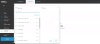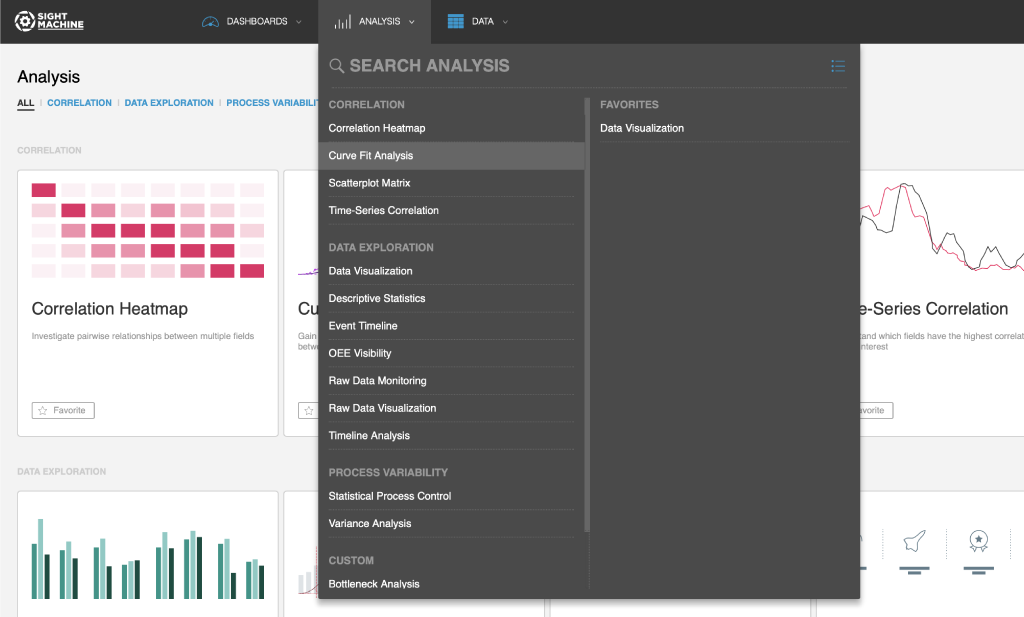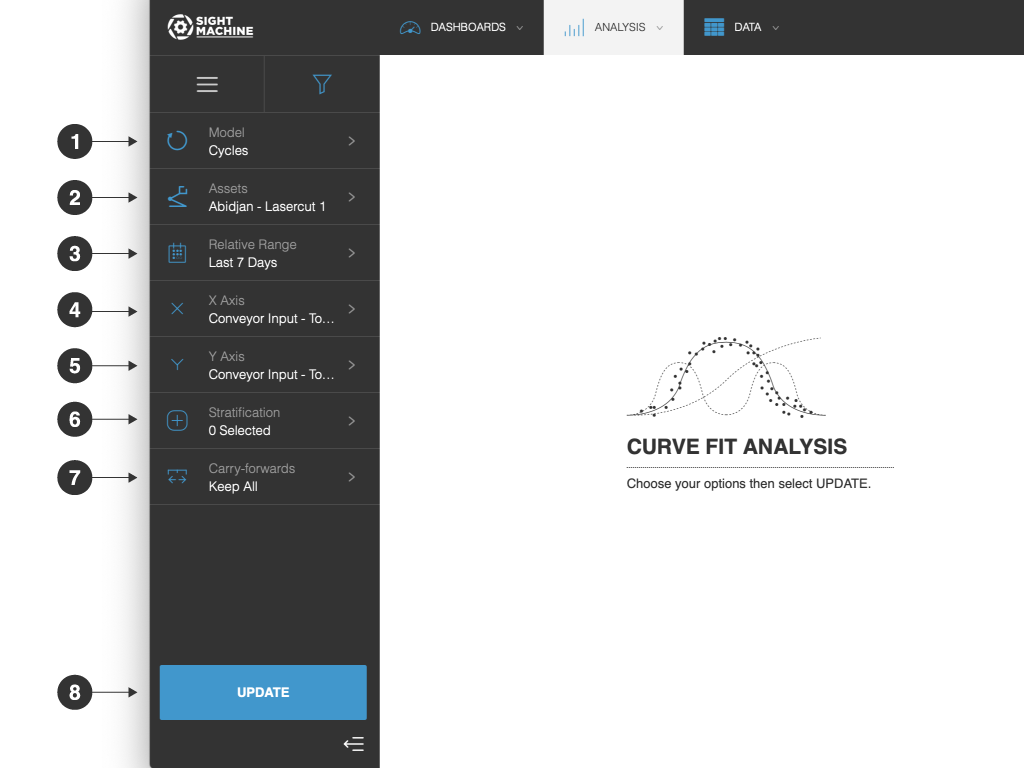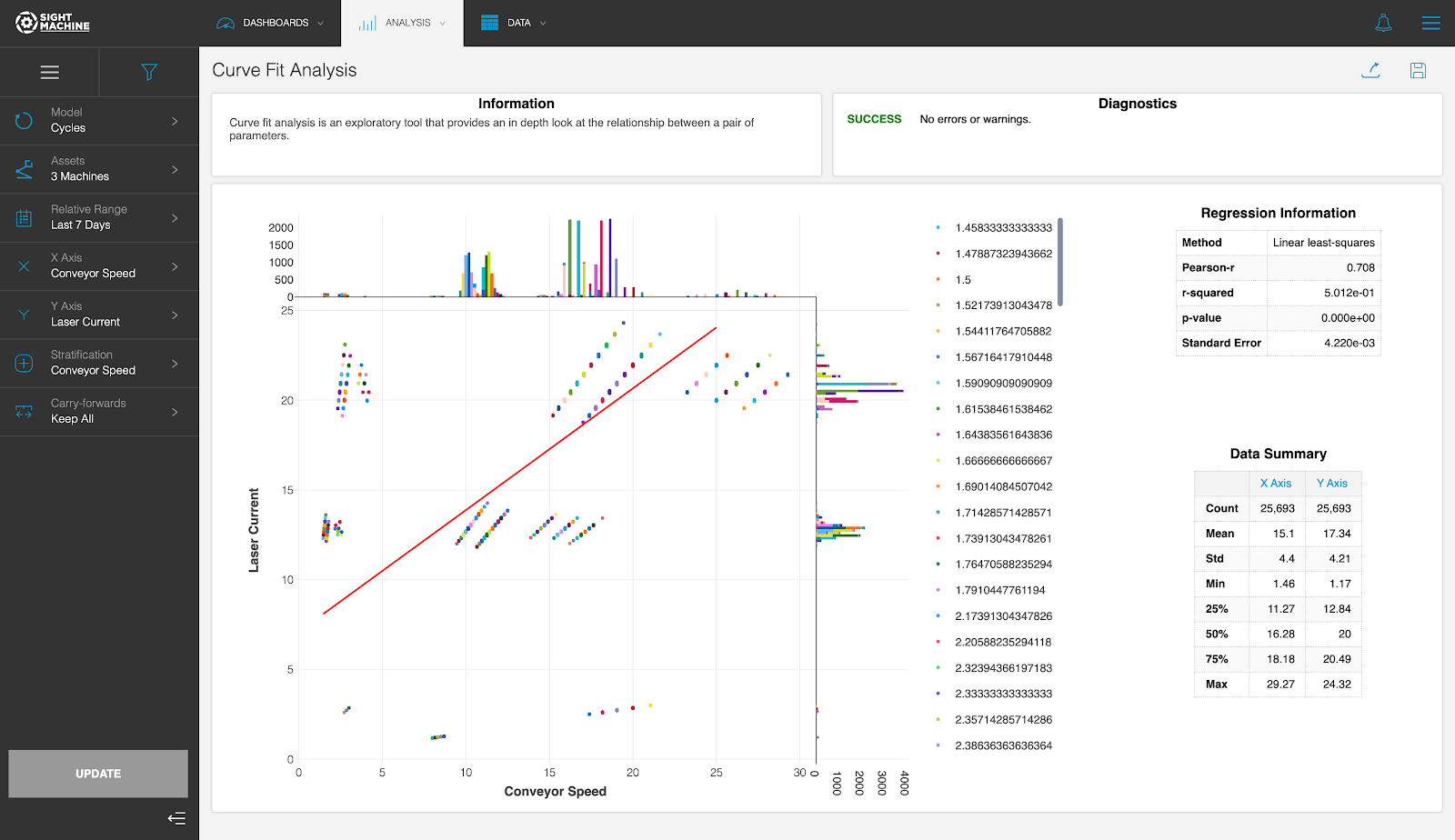# Curve Fit Analysis# Use Cases

The Curve Fit Analysis is designed for investigating the details of the relationship between two parameters. Even though two parameters may have a high correlation value, this may be an artifact of the data shape rather than an indication of a meaningful relationship that can be put to use. The Curve Fit Analysis is most helpful later in the exploratory process, after you have identified a pair of parameters to analyze in detail. This is a good tool to follow the Correlation Heatmap or Time-Series Correlation.

# Specifications

The Curve Fit Analysis is a multivariate analysis that you can perform on continuous fields on the Cycle and Part models. You can select multiple machines of a given type when performing the analysis on the Cycle model. Using the Cycle model, the Curve Fit Analysis can investigate parameters from the same machine type with high granularity. Using the Part model lets you compare fields across different machines, but with lower granularity (the Part model is limited by the serial number or other identifier used to construct the data model).

# Analysis

The Curve Fit Analysis is an exploratory tool that allows you to investigate the relationship between a pair of parameters by computing a linear least-squares regression for the parameters. The analysis plots the selected points on a scatterplot, with a histogram along each axis to show the parameters’ distributions, as well as the fit line determined by the regression. You can choose a categorical field for stratification, which breaks the points into separate traces for each value in the category. The Curve Fit Analysis provides a table of metrics for the regression, including the Pearson-R correlation coefficient and r-squared (see note below). This tool also presents a table of summary statistics for each selected parameter that includes Count, Standard Deviation, Minimum, and Maximum.

NOTE: Statisticians can speak volumes on the finer points of interpreting the r-squared value, but it is basically an estimate of how well the independent variable (the variable on the X axis of your chart) determines the dependent variable (the variable on the Y axis of your chart). Small numbers close to zero indicate a weak relationship. Bigger numbers close to one indicate a stronger relationship.

# How to Use a Curve Fit Analysis

The Curve Fit Analysis tool allows you to take a more in-depth look at the relationship between a pair parameters.

NOTE: This tool only works on numeric fields, not categorical fields such as Pass/Fail.

To use a Curve Fit Analysis:

1. On the Analysis tab, under Correlation, click Curve Fit Analysis.2. On the main Curve Fit Analysis screen, select your options on the left. For more details about each option, see Curve Fit Analysis Options.
3. Click Update.

# Curve Fit Analysis OptionsThe Curve Fit Analysis options include:

1. Model: You can select:
• Cycles: Examine the correlation between two parameters in the same asset or asset type.
• Parts: Examine the correlation between two parameters across different machines producing the same part type.
2. Assets: You can select assets to monitor. For example, to perform a Cycles analysis, you need to select an asset to analyze. You can select 2 specific machines. If you are using the Parts model, you need to select a part type.
3. Relative Range: You can define a date range of data points to analyze for the assets you have selected. For example, select the last 7 days. This option establishes the boundaries for the near real-time data that will be part of your analysis. Select from relative or absolute timeframes.
4. X Axis: You can select a parameter for the asset to be plotted along the X axis.
5. Y Axis: You can select a second parameter for the asset to be plotted along the Y axis.
6. Stratification: You can select a stratification. You can introduce a third dimension by classifying the relationship between data points in different colors for that third parameter. This lets you determine if this third parameter has a relationship with your previously selected parameters. For example, you can select the stratification of machines so that the output is differentiated for each machine.
7. Carry-forwards: You can undo the forward-fill effect on any fields. Select from Keep All, First, or Last. For more information, see the table in Options Common to All Analysis Tools.
8. Update: Click this button to generate your chart.# Tool Output

The Curve Fit tool outputs a scatterplot with a regression line and two histograms, one for each parameter. This enables you to gain a deeper understanding of the relationship between the two parameters.

For example, the stratification that you set applied a different color for the data points for each machine, allowing you to see if the machine impacted the relationship.

The value of correlation is represented by the Pearson-R correlation coefficient, and you can see other useful statistical metrics displayed, such as r-squared (see note below), p-value, and standard error.NOTE: Statisticians can speak volumes on the finer points of interpreting the r-squared value, but it is basically an estimate of how well the independent variable (the variable on the X axis of your chart) determines the dependent variable (the variable on the Y axis of your chart). Small numbers close to zero indicate a weak relationship. Bigger numbers close to one indicate a stronger relationship.